# Free Vibration of Cantilever Beam with Lumped Mass at Free End

2.9 Calculation of damping ratio

The logarithmic decrement is defined as

so that

or

###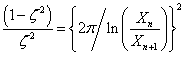Hence, finally we get

###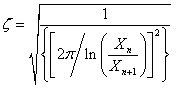(2.15)

Example 2.2: Obtain the damping ratio from the two consecutive peaks of a freely decaying vibration signal as given in table below.

We have,

<

###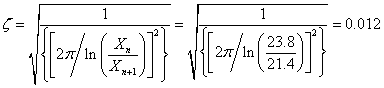2.10 Calculation of experimental natural frequency

Conduct an experiment with the specified cantilever beam specimen to calculate the natural frequency of the cantilever beam experimentally. Record the data of time history (time versus displacement), and plot the graph as shown in Fig. 2.28. Obtain the free vibration response peak values and corresponding time instants.

###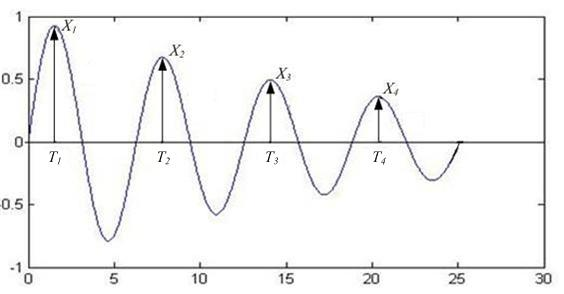Fig. 2.28: Variation of an under-damped free response with time

Let X n is the peak value of the n th peak and Xn +1 is peak value of next consecutive peak and T n  and T n+1 are the corresponding time, respectively. The experimental damped natural frequency is given as,

###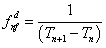(2.16)

and undamped natural frequency is given as,

###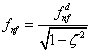(2.17)

Example 2.3: Obtain the damped natural frequency from time instances of two consecutive peaks of a freely decaying signal as given in table below.

 Time ( T 1 ) of the first peak Time ( T 2 ) of the first peak 302 ms 208 ms

Hence, the damped natural frequency is given as

###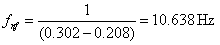(2.18)

and the undamped natural frequency is given as,